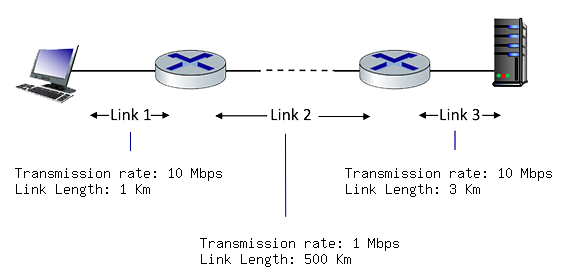# Interactive end-of-chapter exercises

### Computing end-end delay (transmission and propagation delay)

Consider the figure below, with three links, each with the specified transmission rate and link length.Assume the length of a packet is 12000 bits. The speed of light propagation delay on each link is 3x10^8 m/sec